# ISRO Scientist or Engineer Electronics 2013

Instructions

For the following questions answer them individually

Question 1

# Determine the matched filter response h(t) for signal s(t) shown below: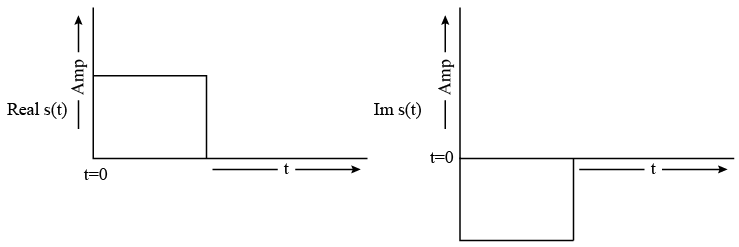Question 2

# A signal source with 100m wavelength is connected to the input terminals of a 150m long transmission line terminated in its characteristic impedance. The phase difference between the voltages at two ends of the transmission line in steady-state condition is :Question 3

# A charge $$Q_2 = 8.854 \times 10^{-9} C$$ is located in a vacuum at $$P_2 (2,3,1)$$. The force on $$Q_2$$ due to a charge $$Q_1 = 4 \pi \times 10^{-3} C$$ at $$P_1 (2,2,1)$$ is: (Note: All the coordinates are measured in Meters. $$a_x, a_y$$, and $$a_z$$ are unit vectors in X, Y and Z direction respectively.)Question 4

# A low pass filter as shown in following figure is built using an operational amplifier having unity gain bandwidth of 1MHz. What is the bandwidth of this circuit ?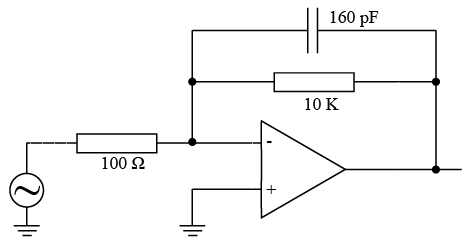Question 5

# What is the frequency and duty cycle of output Y, when CLK frequency is 1 MHz @ 50% duty cycle?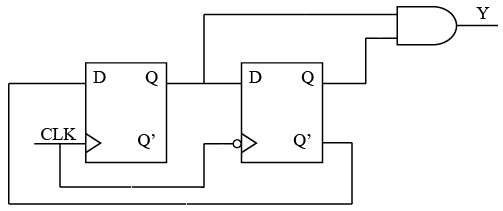Question 6

# For an isotropic radiator, electric field intensity at a distance R is measured as 3 V/m. What will be the electric field intensity at a distance 3R ?Question 7

# The logic function implemented by following 4 :1 MUX is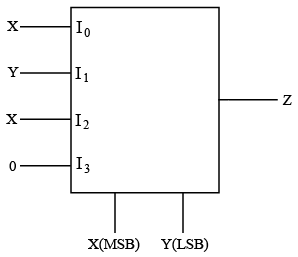Question 8

# Characteristic impedance of a two-wire transmission line at 1OKHz is 200-j50 ohms. Line is terminated in its characteristic impedance, and a 28.28V p-p signal is measured at its input. Determine the real power supplied by the signal source to the line?Question 9

# Which is the correct waveform across capacitor in the following circuit?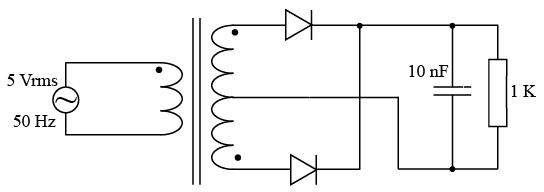Question 10

# Input voltage applied to a circuit is 1V rms and the output is 1 mV rms. Net gain of the circuit is:OR